## Division Algebra

A division algebra, also called a Division Ring or Skew Field, is a Ring in which every Nonzero element has a multiplicative inverse, but multiplication is not Commutative. Explicitly, a division algebra is a set together with two Binary Operatorssatisfying the following conditions:

1. Additive associativity: For all,,

2. Additive commutativity: For all,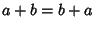,

3. Additive identity: There exists an elementsuch that for all,,

4. Additive inverse: For everythere exists an element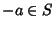such that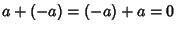,

5. Multiplicative associativity: For all,,

6. Multiplicative identity: There exists an elementnot equal to 0 such that for all,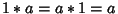,

7. Multiplicative inverse: For everynot equal to 0, there exists,,

8. Left and right distributivity: For all,and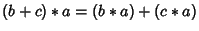.

Thus a division algebrais a Unit Ring for whichis a Group. A division algebra must contain at least two elements. A Commutative division algebra is called a Field.

In 1878 and 1880, Frobenius and Peirce proved that the only associative Real division algebras are real numbers, Complex Numbers, and Quaternions. The Cayley Algebra is the only Nonassociative Division Algebra. Hurwitz (1898) proved that the Algebras of Real Numbers, Complex Numbers, Quaternions, and Cayley Numbers are the only ones where multiplication by unit vectors'' is distance-preserving. Adams (1956) proved that-D vectors form an Algebra in which division (except by 0) is always possible only for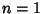, 2, 4, and 8.

References

Dickson, L. E. Algebras and Their Arithmetics. Chicago, IL: University of Chicago Press, 1923.

Dixon, G. M. Division Algebras: Octonions, Quaternions, Complex Numbers and the Algebraic Design of Physics. Dordrecht, Netherlands: Kluwer, 1994.

Herstein, I. N. Topics in Algebra, 2nd ed. New York: Wiley, pp. 326-329, 1975.

Hurwitz, A. Ueber die Composition der quadratischen Formen von beliebig vielen Variabeln.'' Nachr. Gesell. Wiss. Göttingen, Math.-Phys. Klasse, 309-316, 1898.

Kurosh, A. G. General Algebra. New York: Chelsea, pp. 221-243, 1963.

Petro, J. Real Division Algebras of Dimension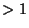contain.'' Amer. Math. Monthly 94, 445-449, 1987.